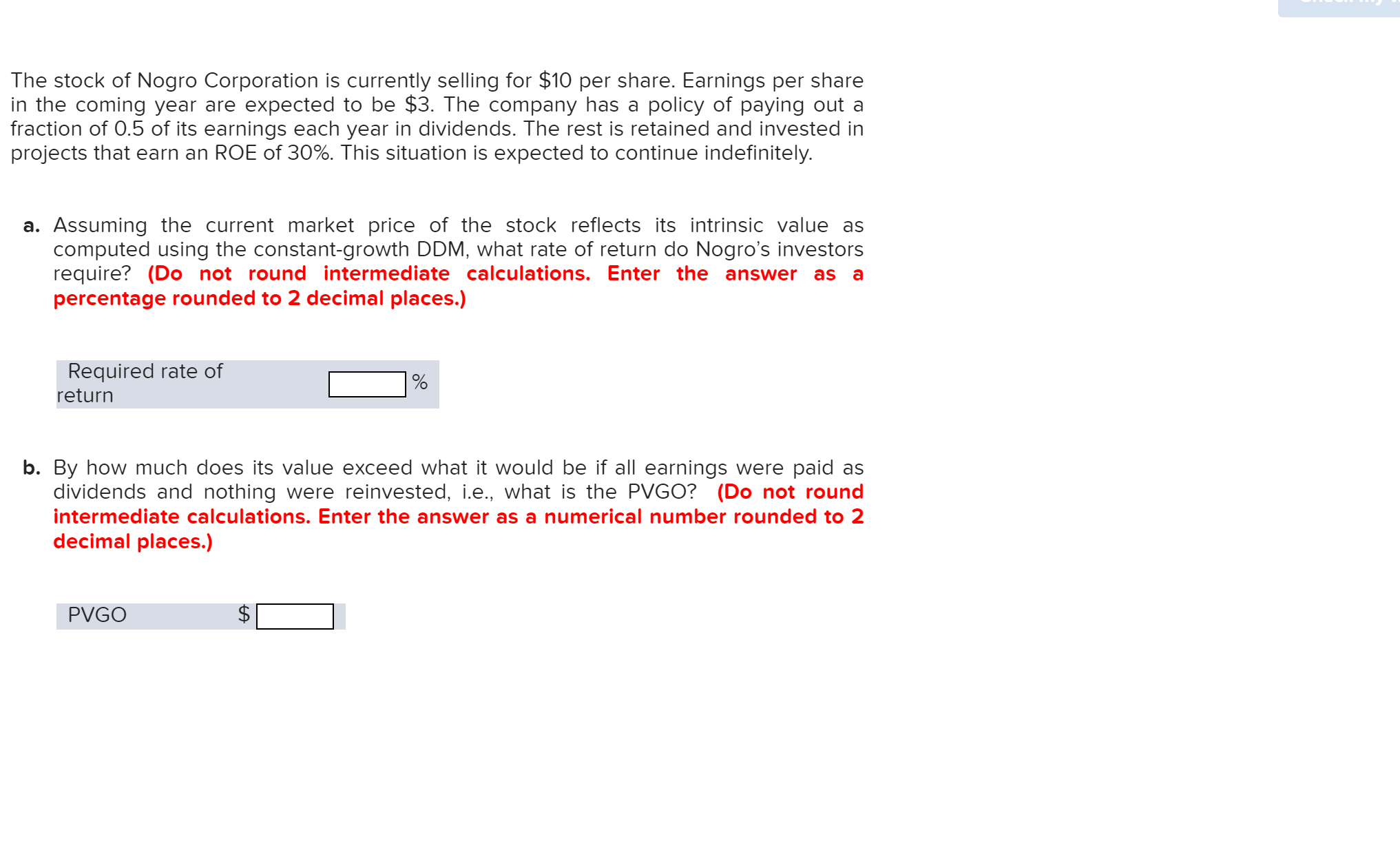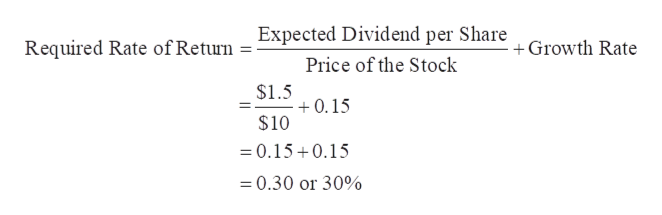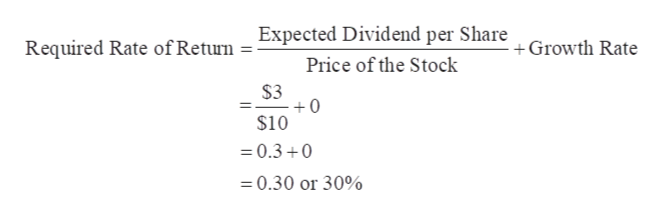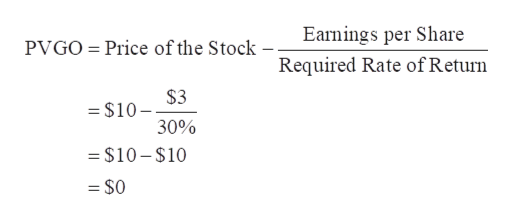# The stock of Nogro Corporation is currently selling for \$10 per share. Earnings per sharein the coming year are expected to be \$3. The company has a policy of paying out afraction of 0.5 of its earnings each year in dividends. The rest is retained and invested inprojects that earn an ROE of 30%. This situation is expected to continue indefinitely.a. Assuming the current market price of the stock reflects its intrinsic value ascomputed using the constant-growth DDM, what rate of return do Nogro's investorsrequire? (Do not round intermediate calculations. Enter the answerpercentage rounded to 2 decimal places.)asRequired rate ofreturnb. By how much does its value exceed what it would be if all earnings were paid asdividends and nothing were reinvested, i.e., what is the PVGO? (Do not roundintermediate calculations. Enter the answer as a numerical number rounded to 2decimal places.)PVGOtA

Question
65 views

need help on allhelp_outlineImage TranscriptioncloseThe stock of Nogro Corporation is currently selling for \$10 per share. Earnings per share in the coming year are expected to be \$3. The company has a policy of paying out a fraction of 0.5 of its earnings each year in dividends. The rest is retained and invested in projects that earn an ROE of 30%. This situation is expected to continue indefinitely. a. Assuming the current market price of the stock reflects its intrinsic value as computed using the constant-growth DDM, what rate of return do Nogro's investors require? (Do not round intermediate calculations. Enter the answer percentage rounded to 2 decimal places.) as Required rate of return b. By how much does its value exceed what it would be if all earnings were paid as dividends and nothing were reinvested, i.e., what is the PVGO? (Do not round intermediate calculations. Enter the answer as a numerical number rounded to 2 decimal places.) PVGO tA fullscreen
check_circle

Step 1

a.

Price of the Stock = \$10

Expected Earnings per Share = \$3

Dividend Pay-out Ratio = 0.5

Expected Dividend per Share = \$3 × 0.5 = \$1.5

Plowback Ratio = 1 – 0.5 = 0.5

ROE = 30%

Growth Rate = 0.5 × 30% = 0.15

Calculation of Required Rate of Return is as follows:help_outlineImage TranscriptioncloseExpected Dividend per Share Required Rate of Return Growth Rate Price of the Stock \$1.5 0.15 \$10 =0.150.15 0.30 or 30% fullscreen
Step 2

b.

Price of the Stock = \$10

Expected Earnings per Share = \$3

Dividend Pay-out Ratio = 100% or 1

Expected Dividend per Share = \$3

Plowback Ratio = 1 – 1 = 0

ROE = 30%

Growth Rate = 0 × 30% = 0

Calculation of Required Rate of Return is as follows:help_outlineImage TranscriptioncloseExpected Dividend per Share Required Rate ofReturn +Growth Rate Price of the Stock \$3 0 \$10 0.3 0 =0.30 or 30% fullscreen
Step 3

Price of the Stock = \$10

Expected Earnings per Share = \$3

Required Rate of Ret...help_outlineImage TranscriptioncloseEarnings per Share Price of the Stock - PVGO Required Rate of Return \$3 = \$10 30% = \$10-\$10 = \$0 fullscreen

### Want to see the full answer?

See Solution

#### Want to see this answer and more?

Solutions are written by subject experts who are available 24/7. Questions are typically answered within 1 hour.*

See Solution
*Response times may vary by subject and question.
Tagged in